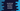# JavaScript parseInt function example to convert a string to integer## JavaScript parseInt function example to convert a string to integer:

In this post, we will learn how to use the parseInt function in JavaScript. The parseInt() function can be used to parse a string and it converts that string to an integer. This method converts the string to integer and returns that value.

### Definition of parseInt:

The parseInt method is defined as like below:

``````parseInt(str)

Here,

• str is the first argument, i.e. the string value to parse. If the argument is not a string, it is converted to a string. Internally, ToString method is used to convert that parameter to a string. If there are any leading whitespaces, the argument is ignored.
• The second parameter, radix is an optional value. It is the base to do the conversion. We can provide a value between 2 to 36. If the provided value is not a number, it will be converted to a number.

### Return value of parseInt:

The parseInt method returns the integer value parsed. If the parse is failed or if any invalid radix is passed, it will return NaN.

### Examples of parseInt:

Let’s take a look at the below example:

``````let arrayOfStrings = [
"12",
"13",
"13.4",
"   12",
"   13   ",
"   13xxy",
"xxy12",
"+12",
"   +13",
"   -14  ",
"-16.7",
];

arrayOfStrings.forEach((i) => console.log(`\${i} => \${parseInt(i)}`));``````

It will print:

``````12 => 12
13 => 13
13.4 => 13
12 => 12
13    => 13
13xxy => 13
xxy12 => NaN
+12 => 12
+13 => 13
-14   => -14
-16.7 => -16``````

As you can see here,

• It returns NaN if the string starts with any character.
• If there is any blank spaces, or +, -, it ignores.
• Since we are not providing the radix value, it takes it as 10.

### Examples of parseInt with hexadecimal string:

If we pass any string starts with 0x and if we don’t provide a radix value, it will take it as a hexadecimal value, i.e. radix as 16.

``````let arrayOfStrings = [
"0x12",
"0xAE8"
];

arrayOfStrings.forEach((i) => console.log(`\${i} => \${parseInt(i)}`));``````

It will print:

``````0x12 => 18
0xAE8 => 2792``````

If we provide 16 as the radix value, it will print the same output.

``````let arrayOfStrings = [
"0x12",
"0xAE8"
];

arrayOfStrings.forEach((i) => console.log(`\${i} => \${parseInt(i, 16)}`));``````